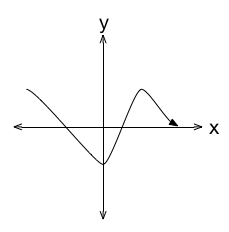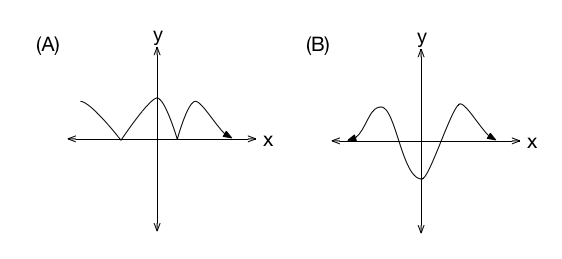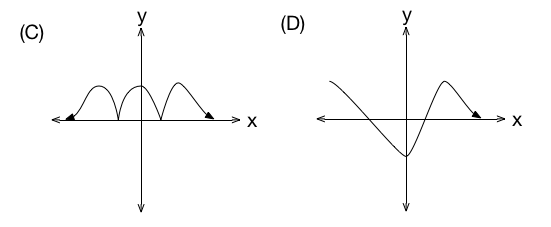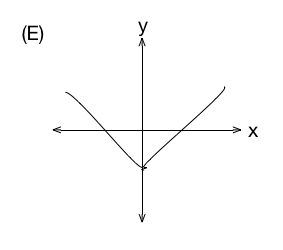# SAT Absolute Value

Algebra Level 3Shown above is the graph of function $y = f(x)$. Which of the following is a graph of the function $y = f( |x| )$?×